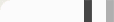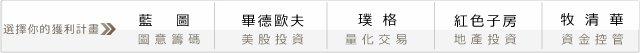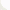## 自適性指標設計(Adaptive Index Design)~ 歷史的確會重演，只是不會很精確地重演 ~

1. 建立市場活性與參數的對應公式或參數映射表 ( 市場活性如：月成交量的變化、月波動率的增減差異值 )，直接將參數與市場活性對應建立一對一或多對一映射表格，根據目前運算出來的市場活性映射出合理參數，再將其帶入原本指標中。代表系統有著名的 DBOII ( The_Dynamic_Break_Out_II Strategy )。2. 布林通道法 ( 波動率 )

BB_H = sma ( ema ( high , n1 ) , n2 ) + 2 * Stddev ( ema ( high , n1 ) , n2 )

BB_L = sma ( ema ( low , n1 ) , n2 ) - 2 * Stddev ( ema ( low , n1 ) , n2 )

3. 參數自動調變

n2 = n1 * ( Stddev ( vol , T2 ) / Stddev ( vol , T1 ) ) , vol 為成交量

n2 = n1 * ( ATR ( close , T2 ) / ATR ( close , T1 ) ) , close 為收盤價

4. 撒尿牛丸法

SuperMA = Power ( SMA * JMA * EMA * HMA * TMA , 1/5 ) ;

SuperMA = ( SMA + JMA + EMA + HMA + TMA ) / 5 ;

5. 回授法 ( Feeadback )

Y(t) = a * Y ( t - 1 ) + b(t)

6. 多資料序列法《 本文由 PROG 璞格交易團隊提供 》

#### 0 意見: